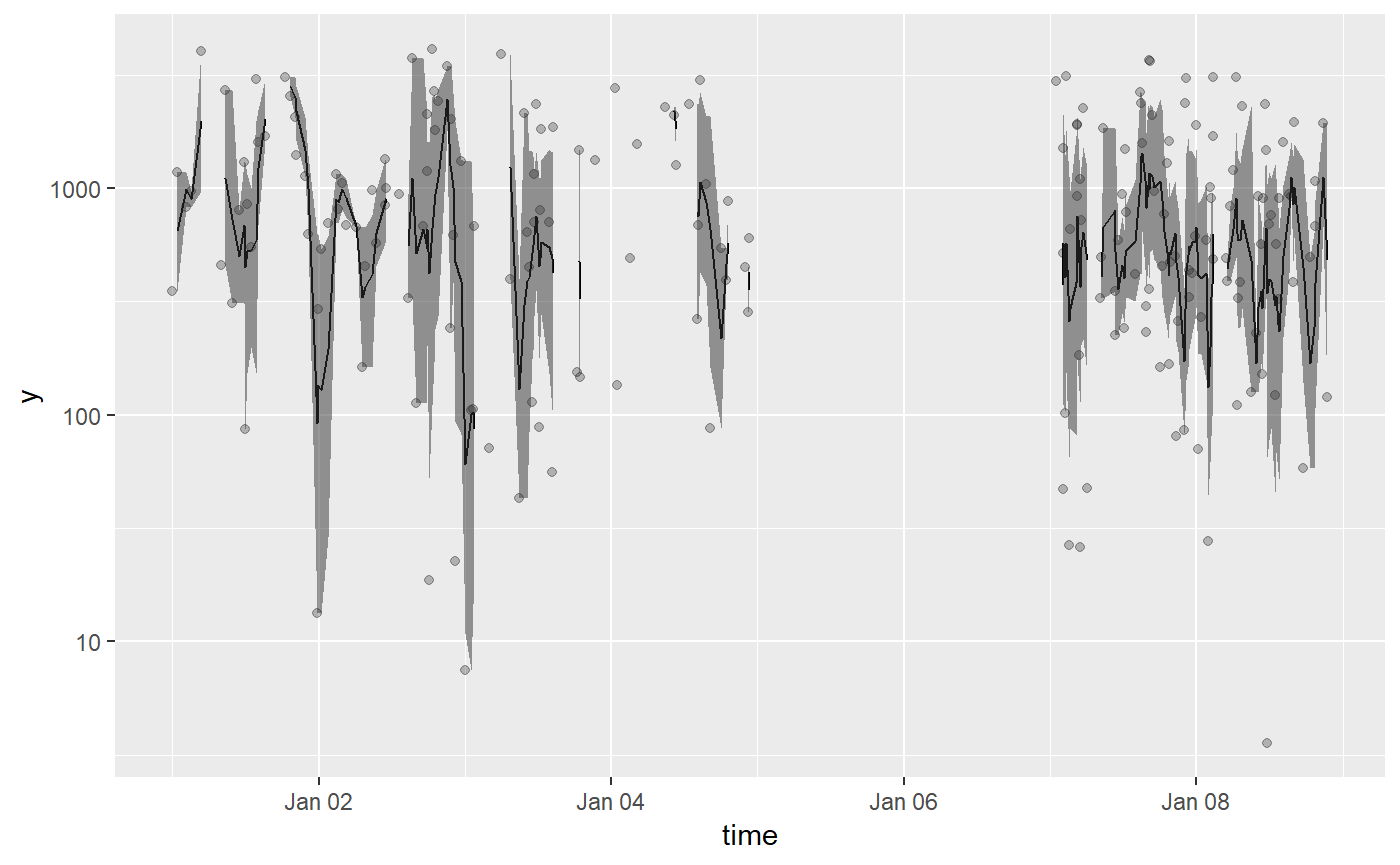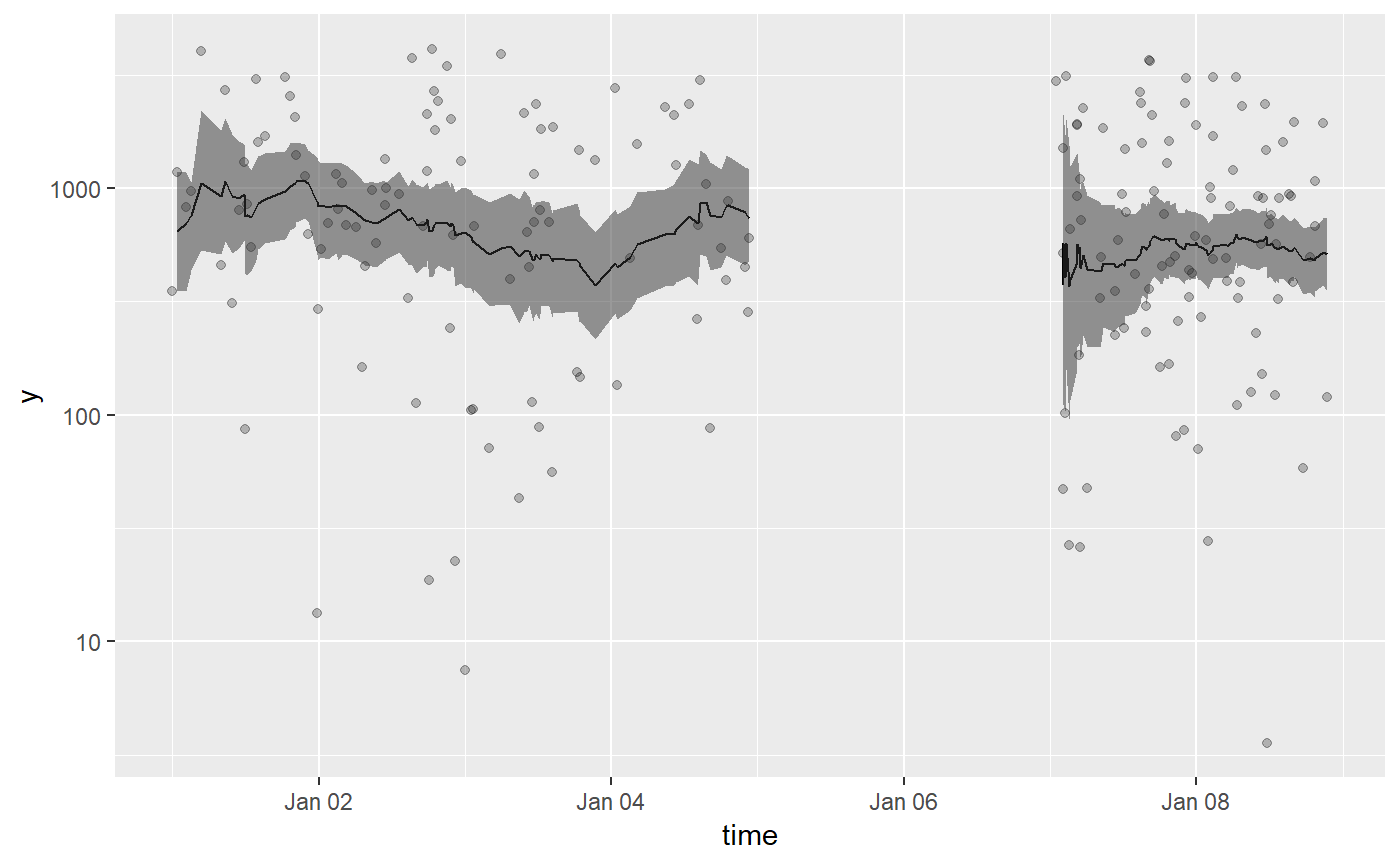The goal of tbrf is to provide time-window based rolling statistical functions. The package differs from other rolling statistic packages because the intended use is for irregular measured data. Althogh tbrf can be used to apply statistical functions to regularly sampled data, zoo, RcppRoll, and other packages provide fast, efficient, and rich implementations of rolling/windowed functions.

An appropriate example case is water quality data that is measured at irregular time intervals. Regulatory compliance is often based on a statistical average measure or exceedance probability applied to all samples collected in the previous $$n$$-years. For each row of data, tbrf functions select previous observations in the time windows specified by the user and applies the statistical function.

Installation

tbrf is available on CRAN:

install.packages("tbrf")

The development version is maintained on github and can be installed as:

devtools::install.github("mps9506/tbrf")

Available Functions

• tbr_binom: Rolling binomal probability with confidence intervals.

• tbr_gmean: Rolling geometric mean with confidence intervals.

• tbr_mean: Rolling mean with confidence intervals.

• tbr_median: Rolling median with confidence intervals.

• tbr_misc: Accepts user specified function.

• tbr_sd: Rolling standard deviation.

• tbr_sum: Rolling sum.

Usage

Core functions include five arguments:

.tbl = dataframe used by the function

x = column containing the values to calculate the statistic on

tcolumn = formatted date-time or date column

unit = character indicating the time unit used, one of "years", "months", "weeks", "days", "hours", "minutes", "seconds"

n = numeric, indicating the window length

Additional arguments for calculating confidence intervals in tbr_gmean, tbr_mean, and tbr_median are passed to boot and boot.ci.

Basic Example

For example, get the 5-year rolling mean:

tbr_mean(Dissolved_Oxygen, x = Average_DO,
tcolumn = Date, unit = "years", n = 5)
## # A tibble: 236 x 9
##    Station_ID Date       Param_Code Param_Desc     Average_DO Min_DO  mean
##         <int> <date>     <chr>      <chr>               <dbl>  <dbl> <dbl>
##  1      12515 2000-01-03 00300      OXYGEN, DISSO~       6.19   6.19 NA
##  2      12515 2000-03-14 00300      OXYGEN, DISSO~       6.7    6.7   6.73
##  3      12517 2000-03-14 00300      OXYGEN, DISSO~       7.3    7.3   6.73
##  4      12515 2000-03-16 00300      OXYGEN, DISSO~       6.41   6.41  6.65
##  5      12515 2000-05-03 00300      OXYGEN, DISSO~       4.42   4.42  6.20
##  6      12517 2000-06-14 00300      OXYGEN, DISSO~       5.74   5.74  6.13
##  7      12515 2000-06-15 00300      OXYGEN, DISSO~       4.86   4.86  5.95
##  8      12515 2000-07-11 00300      OXYGEN, DISSO~       4.48   4.48  5.76
##  9      12515 2000-09-12 00300      OXYGEN, DISSO~       5.64   5.64  5.75
## 10      12517 2000-10-17 00300      OXYGEN, DISSO~       7.96   7.96  5.97
## # ... with 226 more rows, and 2 more variables: lwr_ci <lgl>, upr_ci <lgl>

This works in a tidy-workflow as:

library(ggalt) #for stat="stepribbon""

Dissolved_Oxygen %>%
mutate(Station_ID = as.factor(Station_ID)) %>%
group_by(Station_ID) %>%
tbr_mean(Average_DO, Date, "years", 5, conf = 0.95, type = "perc") %>%
ggplot() +
geom_step(aes(Date, mean, color = Station_ID)) +
geom_ribbon(aes(Date, ymin = lwr_ci, ymax = upr_ci, fill = Station_ID),
stat = "stepribbon", alpha = 0.5)Different Time Units

tbrf works with times or dates.

## Generate some sample data

sampledata <- function(N, start,  end) {
start <- as.POSIXct(start, "%Y-%m-%d %H:%M:%S", tz = "")
end <- as.POSIXct(end, "%Y-%m-%d %H:%M:%S", tz = "")
time <- sample(seq(start, end, by = "min"), N)

df <- tibble(time = time,
y = -1000*log(runif(N)))
return(df)
}

df <- bind_rows(sampledata(100, "2017-01-01 00:01:00", "2017-01-04 23:00:00"),
sampledata(100, "2017-01-07 00:01:00", "2017-01-08 23:00:00"))

Plot 120-minute geometric means:


df %>%
tbr_gmean(y, time, unit = "minutes", n = 120, conf = 0.95, type = "perc") %>%
ggplot() +
geom_point(aes(time, y), alpha = 0.25) +
geom_line(aes(time, mean)) +
geom_ribbon(aes(time, ymin = lwr_ci, ymax = upr_ci), alpha = 0.5) +
scale_y_log10()Plot 24-hour geometric means:

df %>%
tbr_gmean(y, time, unit = "hours", n = 24, conf = 0.95, type = "perc") %>%
ggplot() +
geom_point(aes(time, y), alpha = 0.25) +
geom_line(aes(time, mean)) +
geom_ribbon(aes(time, ymin = lwr_ci, ymax = upr_ci), alpha = 0.5) +
scale_y_log10()Plot 4-day geometric means:

df %>%
tbr_gmean(y, time, unit = "days", n = 4, conf = 0.95, type = "perc") %>%
ggplot() +
geom_point(aes(time, y), alpha = 0.25) +
geom_line(aes(time, mean)) +
geom_ribbon(aes(time, ymin = lwr_ci, ymax = upr_ci), alpha = 0.5) +
scale_y_log10()Parallel Processing

Confidence Intervals in tbr_gmean, tbr_mean, and tbr median are calculated using boot::boot_ci. If you do not need confidence intervals, calculation times are substantially shorter. parallel, ncores, and cl arguments are passed to boot and can improve computation times. See ?boot for more details on parallel operations. An example for parallel processing in Windows is shown below:

library(microbenchmark)
library(snow)

##benchmark run on a windows Intel I7 2.6Ghz dual core laptop

cl <- makeCluster(2, type = "SOCK")

microbenchmark(
"noCI" = tbr_gmean(Dissolved_Oxygen, Average_DO, Date,
"years", 5),
"single" = tbr_gmean(Dissolved_Oxygen, Average_DO, Date,
"years", 5, R = 1000, conf = .95,
type = "perc"),
"parallel" = tbr_gmean(Dissolved_Oxygen, Average_DO, Date,
"years", 5, R = 1000, conf = .95,
type = "perc", parallel = "snow",
ncpus = 2, cl = cl),
times = 20)
## Unit: milliseconds
##      expr        min         lq       mean     median         uq
##      noCI   356.7044   371.4177   387.9659   379.9838   398.2247
##    single 13797.9694 13925.8277 14316.4646 14182.7591 14565.7968
##  parallel  8080.2448  8127.0989  8264.8726  8169.2343  8380.8470
##         max neval
##    476.9167    20
##  15259.7203    20
##   8778.6763    20

stopCluster(cl)